Next: Bibliography

# Spatial Extrema of Advected Scalars

G. D. McBain
School of Engineering, James Cook University, Townsville, QLD 4811, Australia

This note concerns stationary solutions of the advection-diffusion equation without source or sink terms; for example, the steady-state temperature field in a pure fluid or ideal mixture when viscous dissipation, radiation, work against external forces, the Dufour effect, etc. may be neglected. Multiple advecting flows, such as occur in multicomponent mixtures, are explicitly included.

That no such scalar field can possess a strong relative maximum or minimum at an interior point of its domain of existence follows from the positive role of diffusion in eliminating them and the inability of advection to create them. This is reflected mathematically in the positive tensorial character of the diffusivity and the elliptic nature of the equation. The result is easily deduced from Hopf's Maximum Principle. Here, however, an alternative original and quite different proof is presented which, by avoiding the artifice of a comparison function and using vectorial and tensorial concepts rather than a general calculus of several variables, is, it is hoped, more conducive to physical intuition. The use of vectors also frees the result from any particular coordinate system. Since it adds little extra complexity, an anisotropic diffusivity is considered;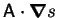being replaced byin the special case of isotropy.

We begin with some definitions.

A divergence-free vector field,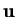, satisfies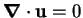.

A positive tensor,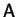, is one for which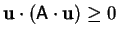for all vectors, with equality implying.

If the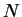scalar functions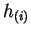and divergence-free vector fields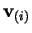and the positive tensor fieldare all continuously differentiable then(1)

is the steady-state advection-diffusion equation for the scalar field,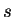.

A regular solution of a partial differential equation is one for which all the partial derivatives occurring in the equation exist and are continuous .

A strong local extremum of a scalar field is a point with a neighbourhood in which the value of the field at every point is greater than at the extremum.

Theorem: No regular solution of the steady-state advection-diffusion equation possesses a strong local extremum.

Proof: The idea for this proof, suggested by Prof. Bob Street (1999, pers. comm., 4 Feb.), is to recast the equation in quasilinear elliptic form, for which the result is known.

Carrying out the divergence,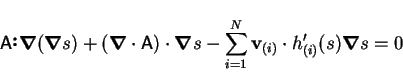(2)

or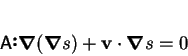(3)

where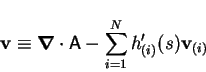(4)

and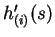is the derivative of.

In Cartesian tensor notation with the summation convention in force, this is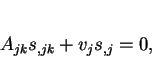(5)

which is of the form for which Hopf's Maximum Principle is shown to hold in treatises on partial differential equations; e.g. Courant and Hilbert . The key here is thatis positive.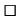Alternative Proof: The proof is by contradiction: assume that there does exist an interior relative extremum. For definiteness, and without loss of generality, take this to be a minimum.

Construct a family of rays originating at the minimum and terminating when they encounter either:

(i)
a boundary point of the domain; or
(ii)
a stationary point, with respect to the ray, of; i.e., where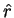is the unit radial vector from the minimum.

Except at the origin, and possibly the rays' termini,is strictly increasing along the rays: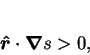(6)

by the definitions of a minimum and the rays (ii). Choose a value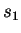ofbetween that at the minimum and the least of those at the rays' termini. Let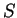be the set of points with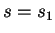passed through by the rays.

Each ray intersectsexactly once, and, sincepossesses at least two continuous spatial derivatives,is closed and smooth enough to have a well-defined unit outward normal,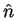. No ray is tangent to, since then the ray should have terminated, by (ii); thus,(7)

Now, by definition of the vector triple product,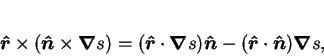(8)

but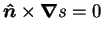, since the normal of a level surface is parallel to the gradient; therefore,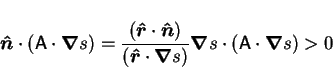(9)

by (6), (7) and sinceis positive. Thus, the inward diffusive flux is positive over the entire surface.

Integrate the steady-state advection-diffusion equation (1) over the volume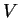enclosed by: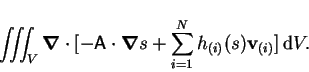(10)

Applying the divergence theorem gives: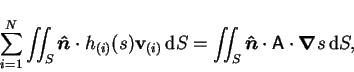(11)

of which the right hand side is positive by (9). The left hand side, however, vanishes;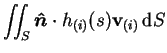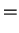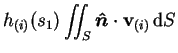(12)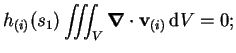(13)

by virtue of the hypotheses on the.

This is a contradiction, so that the theorem is proved.Notes:

• The alternative proof may be summarized as follows. The existence of a strong local extremum would imply the existence of a closed level surface on which the normal component of the gradient, and so the normal component of the diffusive flux, must be of a single sign. Thus, there would always be a net diffusion through the surface, but the net advection would vanish.
• The application to the multicomponent energy equation is clear (cf. ). The variables,,andare the temperature, (tensor) conductivity, partial specific enthalpies and absolute species fluxes, respectively. The required assumption is that the partial specific enthalpies are independent of pressure and composition. The absolute species fluxes are divergence-free because the species they represent are conserved.
• For many common fluids, the diffusivity is isotropic; i.e. a product of a (positive) scalar field and the Kronecker delta; and so is symmetric and positive definite, as required.
• In the special case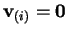and, whereis a constant, the steady-state advection-diffusion equation (1) reduces to Laplace's equation, for which the corresponding result is classical .
• The diffusivity and velocities can depend on, so that the equation is only quasilinear. In the proof,is assumed given, so thatand thecan be re-expressed as functions of position.
• Completely analogous theorems hold in one and two dimensions.
• One can conclude that the temperature minimum apparent in the two-dimensional numerical solutions of Weaver and Viskanta [5,6], and attributed to interdiffusion (the advection of enthalpy by the diffusive flux of a multiple species), was erroneous. The source of the error is the inconsistent treatment of whether the mixture enthalpy did or did not depend on the composition. The sine qua non of interdiffusion is the difference in specific heat capacities of the different species, and interdiffusion only arises from a (frequently convenient) repartitioning of the enthalpy fluxes due to thespecies fluxes into a bulk advective flux and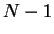interdiffusion fluxes. It is essential, therefore, to treat the mixture enthalpy or specific heat capacity, consistently in the bulk advection and interdiffusion terms. A concise consistent derivation of the energy equation for a binary mixture may be found in reference ; a lengthier discussion has been given elsewhere .
• Extrema might occur if there were source or sink terms in the equations, such as, for the case of the energy equation when the scalar is temperature, one or more of the components changed phase in the domain; the Dufour effect were appreciable; or there were viscous heating.
• Extrema are of course possible in transient advection-diffusion, as for example they may be specified in the initial conditions. An interesting but as yet (to my knowledge) unanswered question is whether strong local extrema can arise in the evolution of a scalar field; this was predicted in the two-dimensional numerical solutions of Bergman and Hyun  for the mass fraction of tin in a nonisothermal amalgam with lead.Next: Bibliography
Geordie McBain
2000-08-10